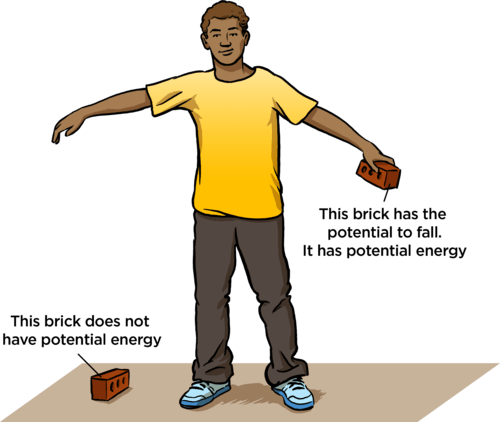Notice: Undefined index: options in /home/u337987201/domains/classnotes.ng/public_html/wp-content/plugins/elementor-pro/modules/theme-builder/widgets/site-logo.php on line 192
Energy - Potential and Kinetic Energy ClassNotes.ng , Forms, Formula.

# Potential and Kinetic Energy

Welcome to Class !!

In today’s Basic Science class, We will be discussing Potential and Kinetic. We hope you enjoy the class!## POTENTIAL AND KINETIC ENERGY

In the previous lesson, we treated potential and kinetic energy briefly as types of mechanical energy under the forms of energy. We shall look into it in details in this lesson.

#### POTENTIAL ENERGY

It is the energy stored in an object due to its position.

Potential energy = mgh

In the above equation, m represents the mass of the object, h represents the height of the object and g represents the acceleration of gravity.

To raise an object m to a height h, we need a work amount equal mgh, and once at this height h, the object has the capability to do work equal to mgh.

An object can store energy as a result of its position. A heavy object lifted up has potential energy due to its position in relation to the ground. It can do work because when dropped, it will fall because of the gravity force, allowing it to make a work output over another object receiving the impact. This stored energy of position is referred to as potential energy.

#####The energy is stored as the result of the gravitational attraction of the earth for the object. The potential energy of the object is dependent on two variables – the mass of the object and the height to which the object is raised. The more massive an object is and the higher that an object is raised, the greater the potential energy.

Potential energy can be stored in elastic materials, such as rubber bands, springs, and an arrow drawn into a bow, as a result of their stretching or compressing. The amount of energy stored in such a material is related to the amount of stretch of the material- the more stretch, the more stored energy. For example, a drawn bow is able to store energy as a result of its position. When not drawn, there is no energy stored in the bow. A compressed spring has potential energy.

For instance, the spring of a mechanical clock transforms its energy doing work to move the seconds, minutes and hour pointers.

There are several kinds of potential energy like gravitational, elastic etc. An object possesses potential energy because of its position with respect to a standard.

## KINETIC ENERGY

It is the energy possessed by an object because of its motion.

Kinetic energy = ½mv2

In the above equation, m represents the mass of the object, v represents the velocity of the object.

The amount of kinetic energy that an object has depends upon two variables: the mass (m) of the object and the speed (v) of the object. An object that has motion- whether it is vertical or horizontal motion- has kinetic energy.There are many forms of kinetic energy- vibrational (the energy due to vibrational motion), rotational (the energy due to rotational motion) and translational (the energy due to the motion from one location to another). The kinetic energy is inherently determined by the speed and mass of the object. This quantity is equal to the work which would be required to bring the body to rest. The kinetic energy does not depend upon the force acting upon the object in a given instant of time. In the example used in the previous lesson, the man pushing the wheelbarrow through a distance converts the chemical energy in his muscles into the kinetic energy of the wheelbarrow.

Now, we can understand the relationship between kinetic and potential energy.

We have come to the end of this class. We do hope you enjoyed the class?

Should you have any further question, feel free to ask in the comment section below and trust us to respond as soon as possible.

In our next class, we will be talking about Calculation involving Work done. We are very much eager to meet you there.

For more class notes, videos, homework help etc download our Mobile App HERE

Join ClassNotes.ng Telegram Community HERE for exclusive content and support

Don`t copy text!